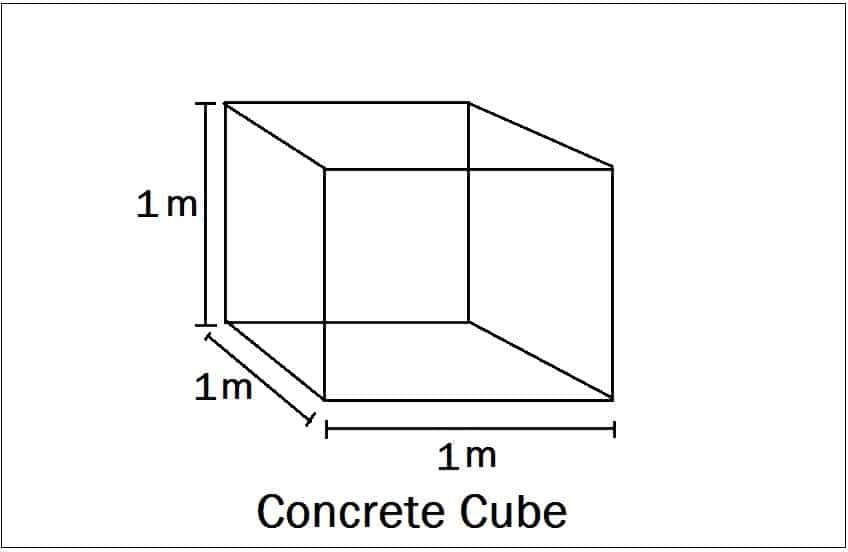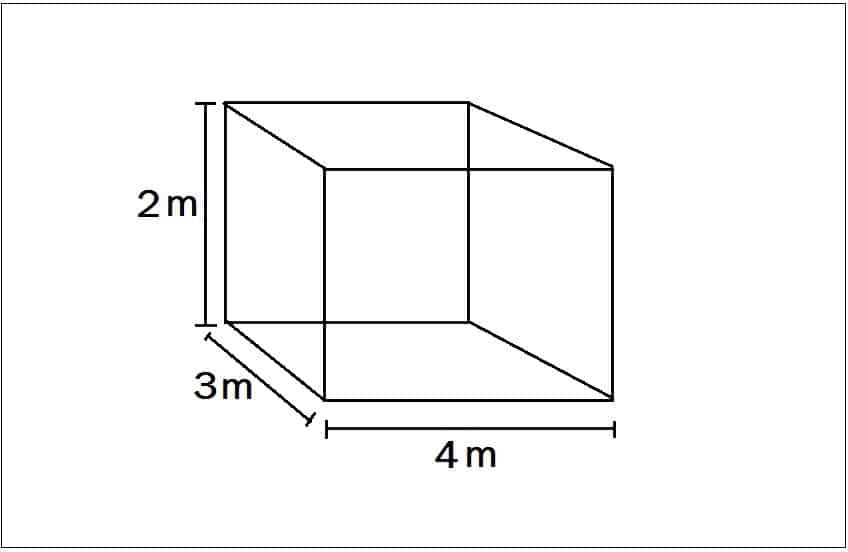# What Is 1.54 In Concrete Calculation

What Is 1.54 In Concrete Calculation?

In concrete calculation, we always multiply Wet volume with 1.54 to get dry volume. But do you know what is 1.54 or where this 1.54 came from? If You don’t know, no problem. In this article, I will explain what is 1.54 while calculating quantity of cement, sand, aggregates for concrete.

let us take a concrete cube.The length of  the concrete cube = 1 m

The width of the concrete cube = 1 m

The height of the concrete cube = 1 m

Volume of concrete cube = length x Width x Height = 1 x 1 x 1 = 1 m³ (Wet volume)

READ -  Common Concreting Problems And Their Prevention

When we convert this we volume into dry volume, the volume is increased by 54% of wet volume.

∴ Dry volume = Wet volume + 54% of Wet volume

= 1 + (54/100) x 1 =1 + 0.54 = 1.54

To convert wet volume of 1 m³ concrete into dry volume = 1.54

To convert wet volume of “n” m³ concrete into dry volume = 1.54 x n

Where n = Wet volume of concrete.

Example:The length of  the concrete cube = 4 m

The width of the concrete cube = 3 m

The height of the concrete cube = 2 m

Wet volume = 4 x 3 x 2 = 24 m³

READ -  Types Of Cement and Their Uses

∴ Dry volume = ( Wet volume + 54% of wet volume)

= 24 + {(54/100) x 24}

= 36.96 m³

Note:

Always remember in concrete calculation, we get wet volume first, to convert wet volume into dry volume, multiply wet volume with 1.54.